# PiGlass

A device that can measure environmental conditions and relay to the user; in glasses form.

IntermediateFull instructions provided4 hours17,377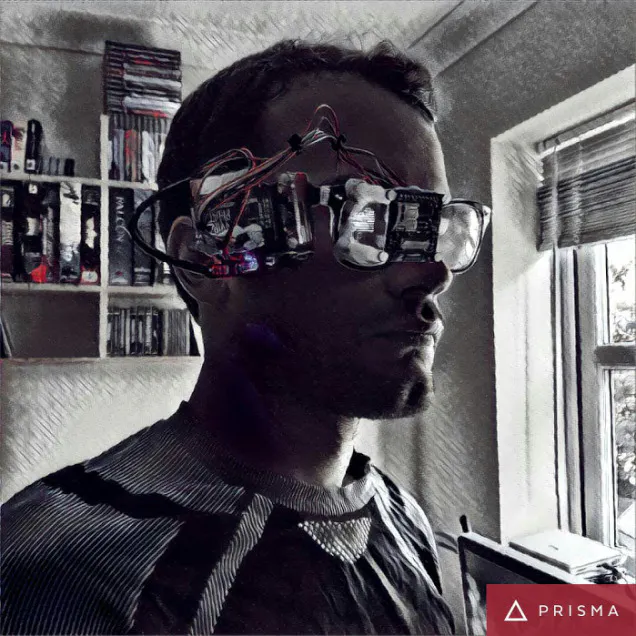## Things used in this project

### Hardware components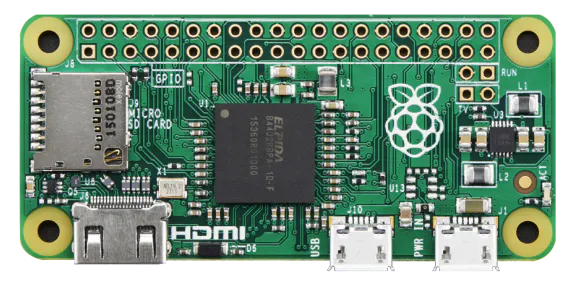Raspberry Pi Zero
×1
 PiBow Zero 1.3
×1
 Geeky Glasses
×1
 Enviro pHAT
×1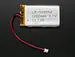Li-Ion Battery 1000mAh
×1
×1
×1
×1
 SD Card
×1
×1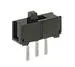Slide Switch
×1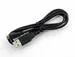USB-A to Micro-USB Cable
×1
 Sugru
×1
 Tie Wraps
×1
 Blu Tak
×1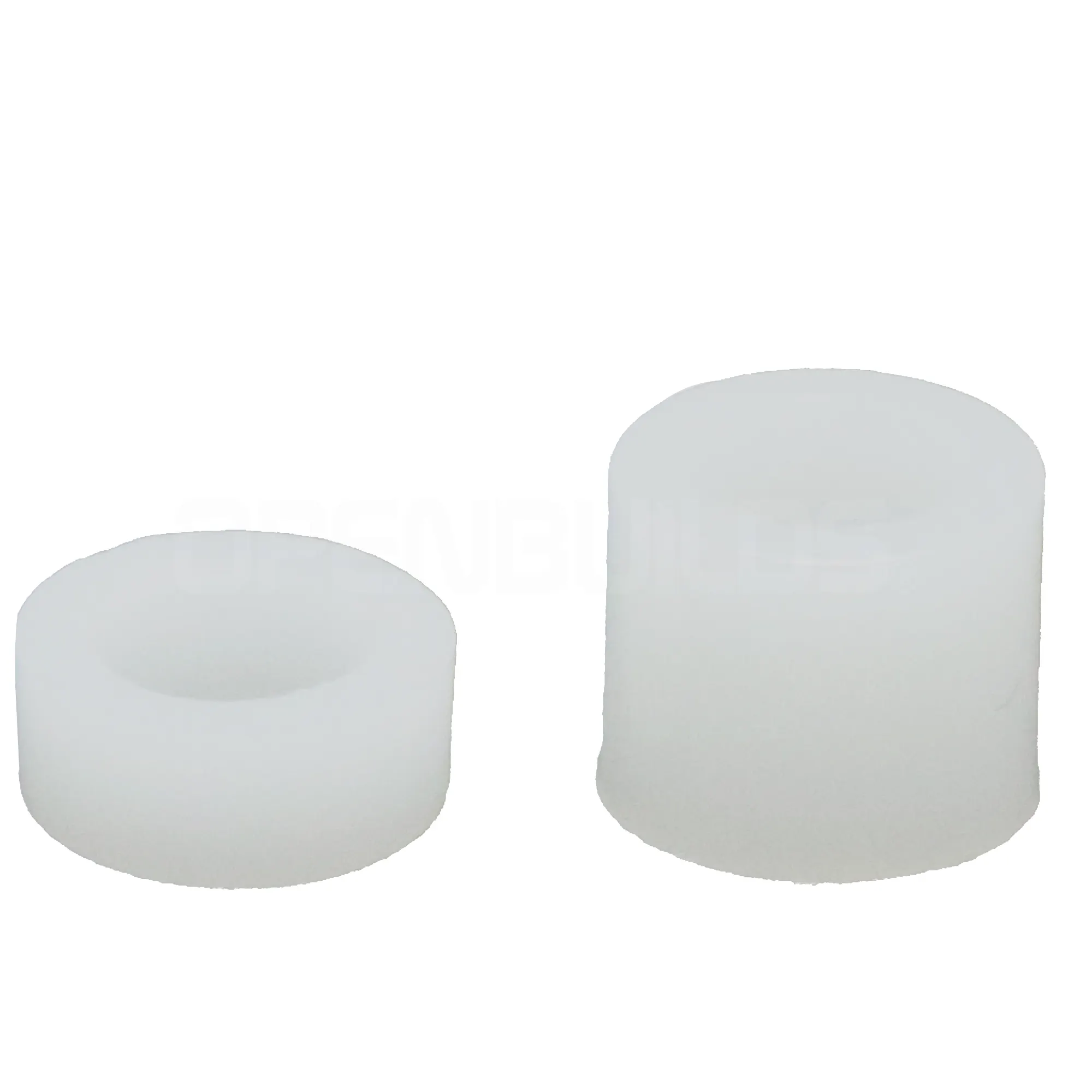OpenBuilds Nylon Spacer
×1
 Nylon Standoffs
×1
 F/F Jumper Wires
×1

### Hand tools and fabrication machinesSoldering iron (generic)

## Code

### Code snippet #1

Plain text
```#imports for the envirophat
from envirophat import light
from envirophat import leds
from envirophat import weather
from envirophat import motion

#general imports
import os
import math
import time
import decimal

#OLED screen imports

#stuff for drawing
import Image
import ImageFont
import ImageDraw

#the setup section for the screen
RST = 24
DC = 23
SPI_PORT = 0
SPI_DEVICE = 0

disp = Adafruit_SSD1351.SSD1351_128_96(rst=RST, dc=DC, spi=SPI.SpiDev(SPI_PORT, SPI_DEVICE, max_speed_hz=8000000))

disp.begin()

width = disp.width
height = disp.height

#determining the direction of north for the heading sensor
north = 294

#main loop
while True:
#setting up the image for the display as well as clearing anything currently on screen
disp.clear()

image = Image.new('1', (width, height))

draw = ImageDraw.Draw(image)

disp.clear()

#here the sensors data is assigned to variables
light_level = light.light()

r, g, b = light.rgb()

temp = weather.temperature()

pressure = weather.pressure()

x, y, z = motion.accelerometer()

#calculating degrees to north

#if the light sensor is covered it will wait 5 seconds, if its still covered after this point, it will shutdown the raspberry pi
if light_level == 0:
time.sleep(5)
light_level = light.light()
if light_level == 0:
os.system("sudo shutdown -h now")
else:
pass

#here i assign headers to the data from the hat, into labelled strings
text_light = ('Light: ' + str(light_level))

text_rgb = ('R: ' + str(r) + ' G: ' + str(g) + ' B: ' + str(b))

text_temp = ('Temp: ' + str(temp))

text_pressure = ('Pressure: ' + str(pressure))

text_motion_1 = ('Motion')

text_motion_2 = ('X: ' + str(x))

text_motion_3 = ('Y: ' + str(y))

text_motion_4 = ('Z: ' + str(z))

#finally the strings are written to the display
draw.text((0, 0), text_light, font=font, fill=255)

draw.text((0, 10), text_rgb, font=font, fill=255)

draw.text((0, 20), text_temp, font=font, fill=255)

draw.text((0, 30), text_pressure, font=font, fill=255)

draw.text((0, 40), text_motion_1, font=font, fill=255)

draw.text((0, 50), text_motion_2, font=font, fill=255)

draw.text((0, 60), text_motion_3, font=font, fill=255)

draw.text((0, 70), text_motion_4, font=font, fill=255)

disp.roughimage(image)

time.sleep(1)
```

### Code snippet #2

Plain text
```#imports for the envirophat
from envirophat import light
from envirophat import leds
from envirophat import weather
from envirophat import motion

#general imports
import os
import math
import time
import decimal

#OLED screen imports

#stuff for drawing
import Image
import ImageFont
import ImageDraw

#the setup section for the screen
RST = 24
DC = 23
SPI_PORT = 0
SPI_DEVICE = 0

disp = Adafruit_SSD1351.SSD1351_128_96(rst=RST, dc=DC, spi=SPI.SpiDev(SPI_PORT, SPI_DEVICE, max_speed_hz=8000000))

disp.begin()

width = disp.width
height = disp.height

#determining the direction of north for the heading sensor
north = 294

#main loop
while True:
#setting up the image for the display as well as clearing anything currently on screen
disp.clear()

image = Image.new('1', (width, height))

draw = ImageDraw.Draw(image)

disp.clear()

#here the sensors data is assigned to variables
light_level = light.light()

r, g, b = light.rgb()

temp = weather.temperature()

pressure = weather.pressure()

x, y, z = motion.accelerometer()

#calculating degrees to north

#if the light sensor is covered it will wait 5 seconds, if its still covered after this point, it will shutdown the raspberry pi
if light_level == 0:
time.sleep(5)
light_level = light.light()
if light_level == 0:
os.system("sudo shutdown -h now")
else:
pass

#here i assign headers to the data from the hat, into labelled strings
text_light = ('Light: ' + str(light_level))

text_rgb = ('R: ' + str(r) + ' G: ' + str(g) + ' B: ' + str(b))

text_temp = ('Temp: ' + str(temp))

text_pressure = ('Pressure: ' + str(pressure))

text_motion_1 = ('Motion')

text_motion_2 = ('X: ' + str(x))

text_motion_3 = ('Y: ' + str(y))

text_motion_4 = ('Z: ' + str(z))

#finally the strings are written to the display
draw.text((0, 0), text_light, font=font, fill=255)

draw.text((0, 10), text_rgb, font=font, fill=255)

draw.text((0, 20), text_temp, font=font, fill=255)

draw.text((0, 30), text_pressure, font=font, fill=255)

draw.text((0, 40), text_motion_1, font=font, fill=255)

draw.text((0, 50), text_motion_2, font=font, fill=255)

draw.text((0, 60), text_motion_3, font=font, fill=255)

draw.text((0, 70), text_motion_4, font=font, fill=255)

disp.roughimage(image)

time.sleep(1)
```

## Credits

### Michael Darby - 314Reactor

32 projects • 83 followers
I like to keep fit, explore and of course make projects. https://314reactor.com/ https://www.facebook.com/314reactor